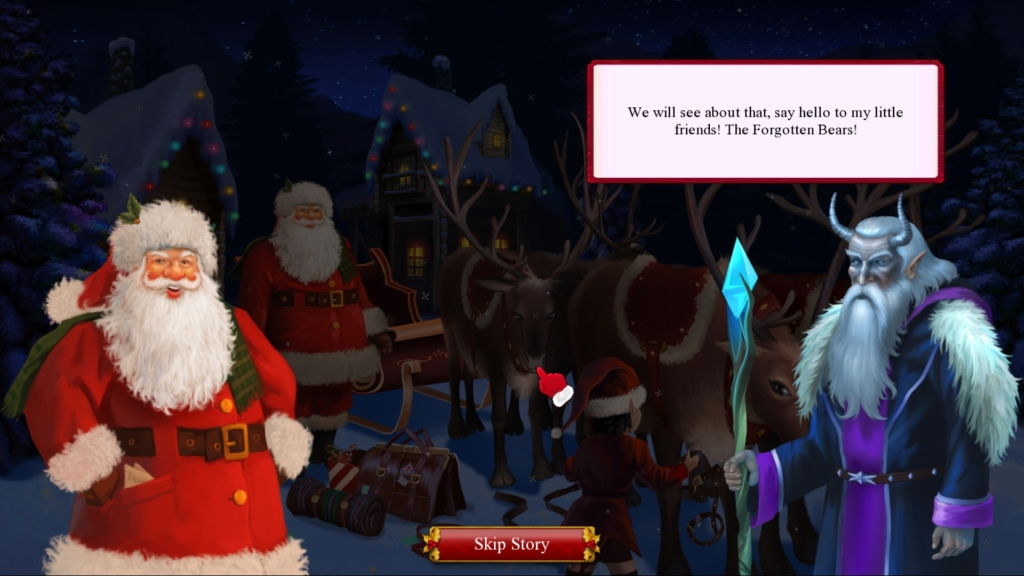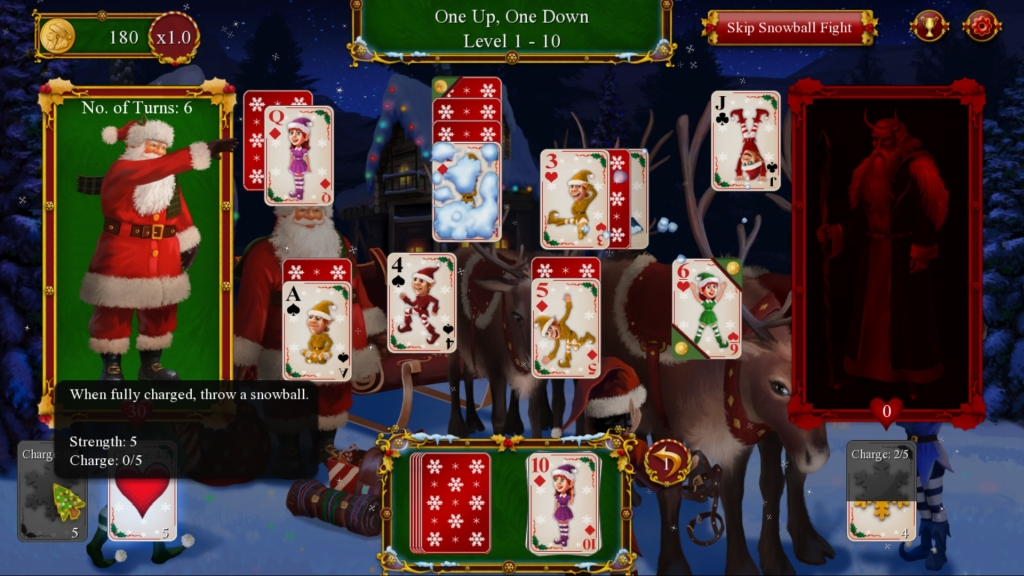-=+=- -=+=- -=+=- -=+=- -=+=- -=+=- -=+=- -=+=- -=+=- -=+=- -=+=- -=+=- -=+=- -=+=- -=+=- -=+=- -=+=- -=+=- -=+=- -=+=- -=+=- -=+=- -=+=- -=+=- -=+=- -=+=- -=+=- -=+=- -=+=- -=+=- (c) WidthPadding Industries 1987 0|651|0 -=+=- -=+=- -=+=- -=+=- -=+=- -=+=- -=+=- -=+=- -=+=- -=+=- -=+=- -=+=- -=+=- -=+=- -=+=- -=+=- -=+=- -=+=- -=+=- -=+=- -=+=- -=+=- -=+=- -=+=- -=+=- -=+=- -=+=- -=+=- -=+=- -=+=-
SoCoder -> Showcase Home -> Puzzle

therevillsgamesCreated : 20 December 2019

System : Windows
Language : Blitz Max

### Santa's Christmas Solitaire 2

Christmas + Solitaire = Santa's Christmas Solitaire 2

Steam
Big Fish Games
ScreenshotsAnother year, another solitaire game! Building from our previous solitaire games we enhanced the game engine to include 3 solitaire types:

* One Up, One Down
* Pair Match
* Sum of 14

Also we have gone back to what made solitaire solitaire! Instead of objectives per hand, it is all about removing cards.

And taking inspiration from Shadowhand, we have included a snowball fight card game in which Santa will do battle with Krampus and his army of teddy bears!

Coded in the classic BlitzMax programming language which has been rock solid for years, Santa's Christmas Solitaire 2 is going to be released on major casual game portals (Big Fish Games, GameHouse etc) and Steam!

Friday, 20 December 2019, 16:35
JayenkaiGreat to see you're still making these, and that BMax is still carrying on!
Good luckFriday, 20 December 2019, 16:51
therevillsgamesThanks JaySaturday, 21 December 2019, 02:45
PakzGood to see blitzmax stil is useful! Monkey2 is now broken on the new macos update from what i gather.

Good luck with the game!